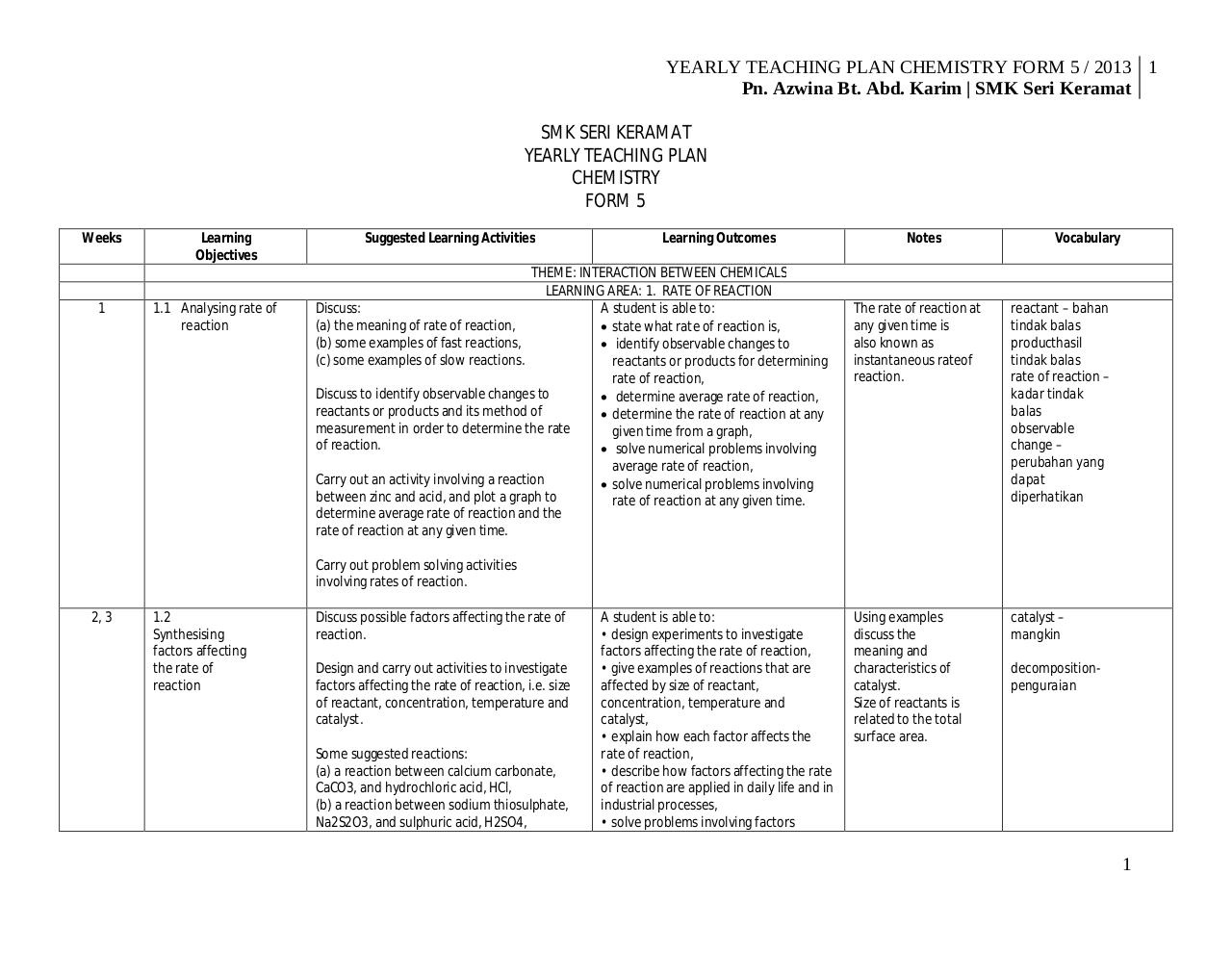# RPT Kimia Tingkatan 5.pdfPage 12320

#### Text preview

YEARLY TEACHING PLAN CHEMISTRY FORM 5 / 2013 1
Pn. Azwina Bt. Abd. Karim | SMK Seri Keramat
SMK SERI KERAMAT
YEARLY TEACHING PLAN
CHEMISTRY
FORM 5
Weeks

1

Learning
Objectives

1.1 Analysing rate of
reaction

Suggested Learning Activities

Learning Outcomes

THEME: INTERACTION BETWEEN CHEMICALS
LEARNING AREA: 1. RATE OF REACTION
Discuss:
A student is able to:
(a) the meaning of rate of reaction,
 state what rate of reaction is,
(b) some examples of fast reactions,
 identify observable changes to
(c) some examples of slow reactions.
reactants or products for determining
rate of reaction,
Discuss to identify observable changes to
 determine average rate of reaction,
reactants or products and its method of
 determine the rate of reaction at any
measurement in order to determine the rate
given time from a graph,
of reaction.
 solve numerical problems involving
average rate of reaction,
Carry out an activity involving a reaction
 solve numerical problems involving
between zinc and acid, and plot a graph to
rate of reaction at any given time.
determine average rate of reaction and the
rate of reaction at any given time.

Notes

Vocabulary

The rate of reaction at
any given time is
also known as
instantaneous rateof
reaction.

reactant – bahan
tindak balas
producthasil
tindak balas
rate of reaction –
balas
observable
change –
perubahan yang
dapat
diperhatikan

Using examples
discuss the
meaning and
characteristics of
catalyst.
Size of reactants is
related to the total
surface area.

catalyst –
mangkin

Carry out problem solving activities
involving rates of reaction.
2, 3

1.2
Synthesising
factors affecting
the rate of
reaction

Discuss possible factors affecting the rate of
reaction.
Design and carry out activities to investigate
factors affecting the rate of reaction, i.e. size
of reactant, concentration, temperature and
catalyst.
Some suggested reactions:
(a) a reaction between calcium carbonate,
CaCO3, and hydrochloric acid, HCl,
(b) a reaction between sodium thiosulphate,
Na2S2O3, and sulphuric acid, H2SO4,

A student is able to:
• design experiments to investigate
factors affecting the rate of reaction,
• give examples of reactions that are
affected by size of reactant,
concentration, temperature and
catalyst,
• explain how each factor affects the
rate of reaction,
• describe how factors affecting the rate
of reaction are applied in daily life and in
industrial processes,
• solve problems involving factors

decompositionpenguraian

1